# ML 108: Evasion Attacks on MNIST dataset (40 pts extra)

## What You Need

• A Web browser

## Purpose

To practice making simple machine learning code in Python. This project is based on this tutorial:

In a browser, go to

From the menu, click File, "New notebook".

Execute these commands to import the SecML library and download the MNIST dataset, which contains 70,000 small images of handwritten digits.
```!pip install git+https://github.com/pralab/secml import secml from secml.data.loader import CDataLoaderMNIST loader = CDataLoaderMNIST() ```
As shown below, a few pages of messages scroll by, ending with several "File stored" messages.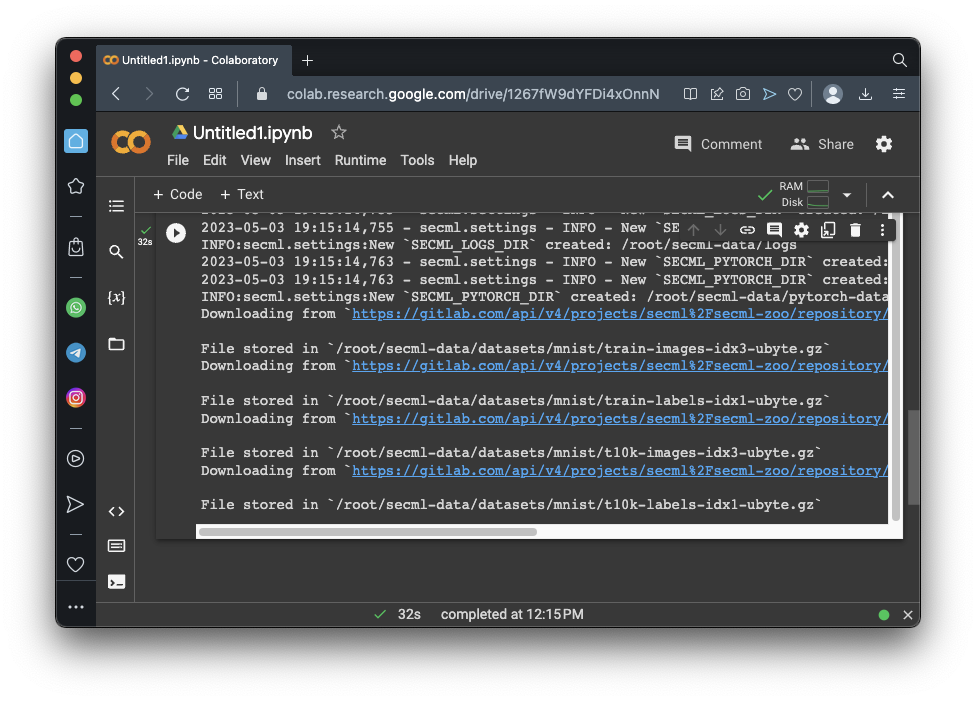## Selecting Samples to Use

Execute these commands to select a small set of samples, including only the digits 5 and 9.
```random_state = 999 n_tr = 100 # Number of training set samples n_val = 500 # Number of validation set samples n_ts = 500 # Number of test set samples digits = (5, 9) tr_val = loader.load('training', digits=digits, num_samples=n_tr + n_val) ts = loader.load('testing', digits=digits, num_samples=n_ts) # Split in training and validation set tr = tr_val[:n_tr, :] val = tr_val[n_tr:, :] # Normalize the features in `[0, 1]` tr.X /= 255 val.X /= 255 ts.X /= 255 print("Training Set") print(tr.X) print(tr.X.shape) print("Validation Set") print(val.X) print(val.X.shape) print("Test Set") print(ts.X) print(ts.X.shape) ```
As shown below, each set is an array of images, each containing 784 pixels (28x28).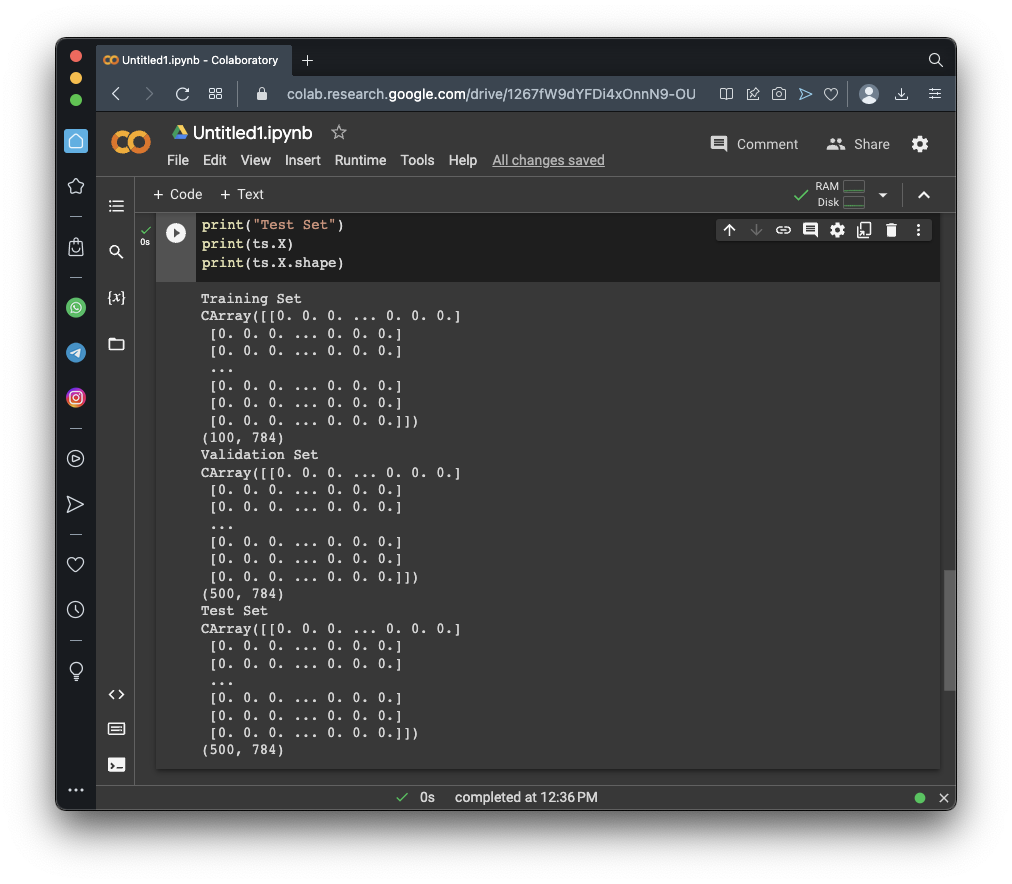## Viewing Samples

Execute these commands to show some examples of the images:
```from secml.figure import CFigure # Only required for visualization in notebooks %matplotlib inline # Let's define a convenience function to easily plot the MNIST dataset def show_digits_1(samples, labels, digs, n_display=8): samples = samples.atleast_2d() n_display = min(n_display, samples.shape) fig = CFigure(width=n_display*2, height=3) for idx in range(n_display): fig.subplot(2, n_display, idx+1) fig.sp.xticks([]) fig.sp.yticks([]) fig.sp.imshow(samples[idx, :].reshape((28, 28)), cmap='gray') fig.sp.title("{}".format(digits[labels[idx].item()])) fig.show() show_digits_1(tr.X, tr.Y, digits) ```
As shown below, the images are handwritten 5's or 9's.## Training a Classifier

Execute these commands to create and train a model:
```from secml.ml.classifiers import CClassifierSVM # train SVM in the dual space, on a linear kernel, as needed for poisoning clf = CClassifierSVM(C=10, kernel='linear') print("Training of classifier...") clf.fit(tr.X, tr.Y) # Compute predictions on a test set y_pred = clf.predict(ts.X) # Metric to use for performance evaluation from secml.ml.peval.metrics import CMetricAccuracy metric = CMetricAccuracy() # Evaluate the accuracy of the classifier acc = metric.performance_score(y_true=ts.Y, y_pred=y_pred) print("Accuracy on test set: {:.2%}".format(acc)) ```
As shown below, the model is 93.6% accurate.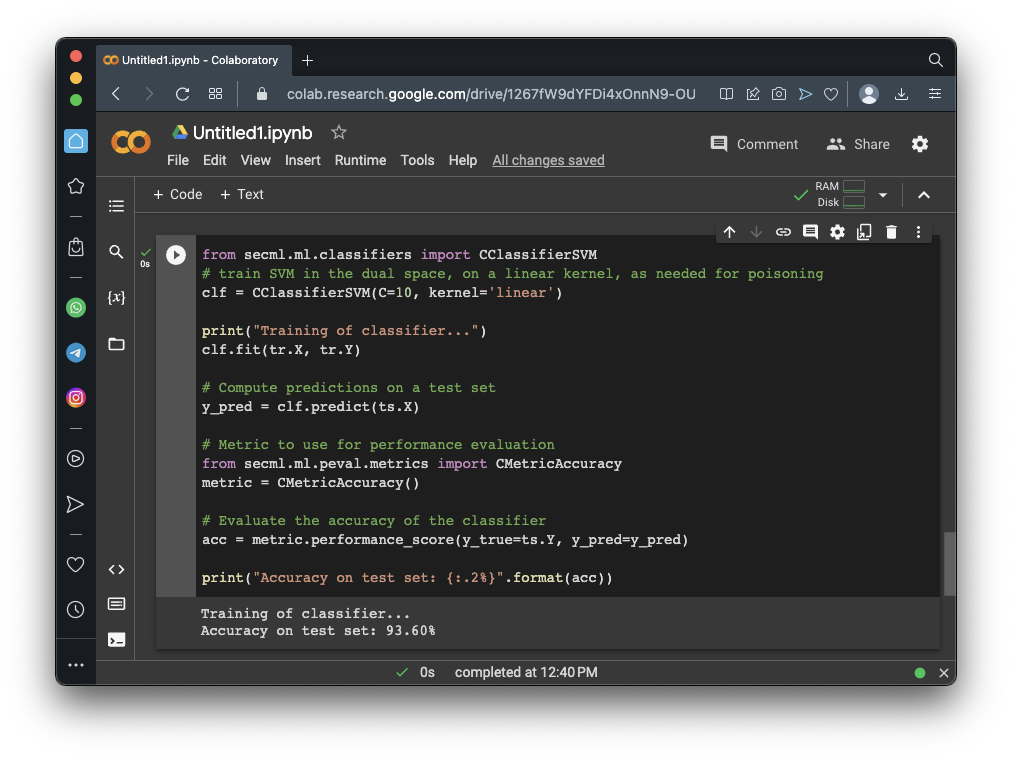## An Evasion Attack

Execute these commands to perform an evasion attack--that is, to modify some of the test images so that the model will make more errors. We'll use only 25 images.
```# For simplicity, let's attack a subset of the test set attack_ds = ts[:25, :] noise_type = 'l2' # Type of perturbation 'l1' or 'l2' dmax = 2.5 # Maximum perturbation lb, ub = 0., 1. # Bounds of the attack space. Can be set to `None` for unbounded y_target = None # None if `error-generic` or a class label for `error-specific` # Should be chosen depending on the optimization problem solver_params = { 'eta': 0.5, 'eta_min': 2.0, 'eta_max': None, 'max_iter': 100, 'eps': 1e-6 } from secml.adv.attacks import CAttackEvasionPGDLS pgd_ls_attack = CAttackEvasionPGDLS(classifier=clf, double_init_ds=tr, distance=noise_type, dmax=dmax, solver_params=solver_params, y_target=y_target) print("Attack started...") eva_y_pred, _, eva_adv_ds, _ = pgd_ls_attack.run(attack_ds.X, attack_ds.Y) print("Attack complete!") acc = metric.performance_score( y_true=attack_ds.Y, y_pred=clf.predict(attack_ds.X)) acc_attack = metric.performance_score( y_true=attack_ds.Y, y_pred=eva_y_pred) print("Accuracy on reduced test set before attack: {:.2%}".format(acc)) print("Accuracy on reduced test set after attack: {:.2%}".format(acc_attack)) ```
As shown below, the model is 100% accurate on these 25 images before the attack, but only 12% accurate afterwards.## Viewing the Modified Images

Execute these commands to show examples of the images before and after the attack:
```from secml.figure import CFigure # Only required for visualization in notebooks %matplotlib inline # Let's define a convenience function to easily plot the MNIST dataset def show_digits(samples, preds, labels, digs, n_display=8): samples = samples.atleast_2d() n_display = min(n_display, samples.shape) fig = CFigure(width=n_display*2, height=3) for idx in range(n_display): fig.subplot(2, n_display, idx+1) fig.sp.xticks([]) fig.sp.yticks([]) fig.sp.imshow(samples[idx, :].reshape((28, 28)), cmap='gray') fig.sp.title("{} ({})".format(digits[labels[idx].item()], digs[preds[idx].item()]), color=("green" if labels[idx].item()==preds[idx].item() else "red")) fig.show() show_digits(attack_ds.X, clf.predict(attack_ds.X), attack_ds.Y, digits) show_digits(eva_adv_ds.X, clf.predict(eva_adv_ds.X), eva_adv_ds.Y, digits) ```
The results are shown below. Note these things:
• The top row shows the original images.
• The numbers above the images are green when the prediction was correct, and red when it was incorrect.
• All the predictions in the top row are correct.
• The bottom row shows the modified images.
• All the visible predictions in the bottom row are incorrect.
Very small changes to the images, hardly noticable, greatly degrade the model's performance. This is a big, systematic problem with machine learning.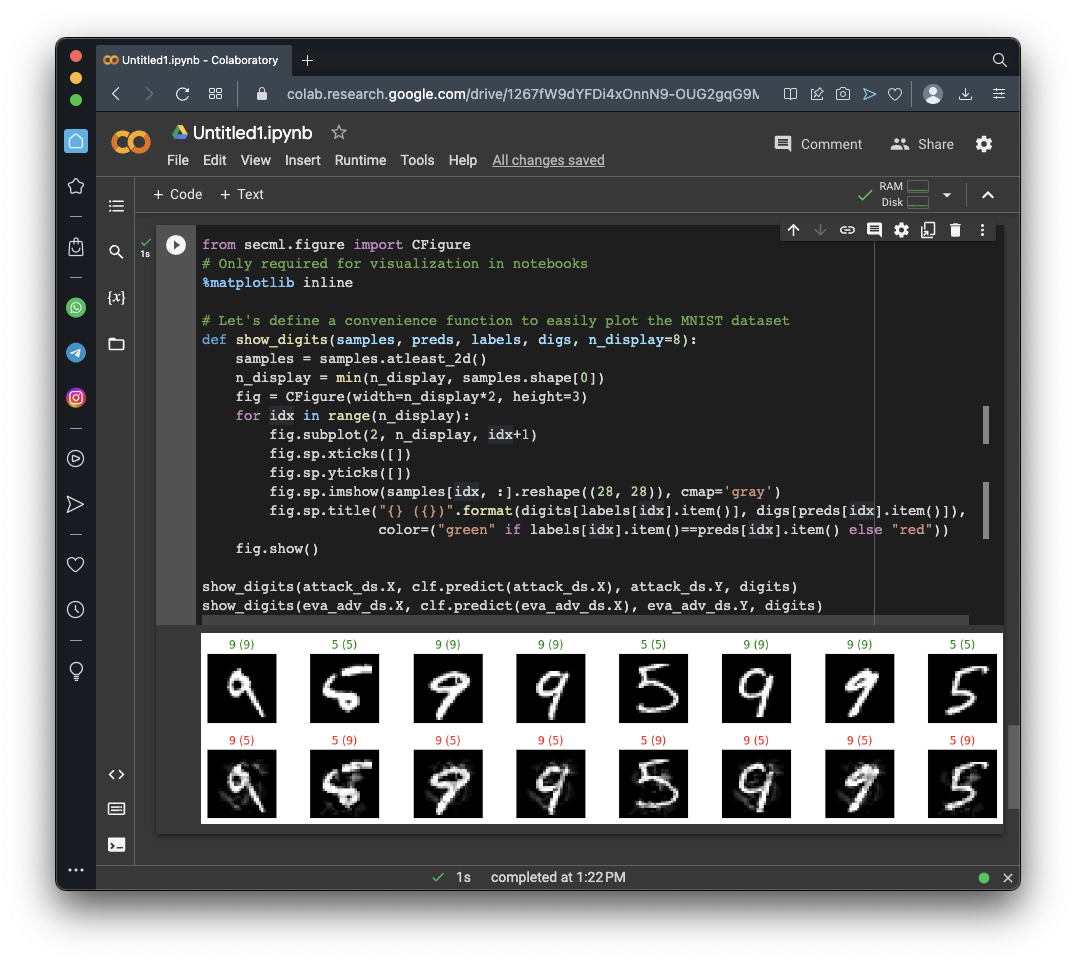## Flag ML 108.1: Correct Predictions (10 pts)

Modify the code above to show all 25 images, or, even better, to print the labels in the second row separate from the images.

Find all the correct predictions in the bottom row and concatenate their values. For example, if there are only three correct, and they are all 9's, the result is 999.

That concatenated number is the flag.

## Flag ML 108.2: Smaller Perturbation (10 pts)

Make these changes:
• Change the maximum perturbation to 2.0
• Change the maximum iterations to 50
The modified images have different patterns of blurry smudges added, as shown below.As in flag 108.1, find all the correct predictions in the 25 images in the bottom row and concatenate their values to form the flag.

## Flag ML 108.3: Different Perturbation Type (10 pts)

Make these changes:
• Change the perturbation type to 11
• Change the maximum perturbation to 12.0
• Change the maximum iterations to 40
The modified images now have only bright or dark pixels added, rather than grays, as shown below.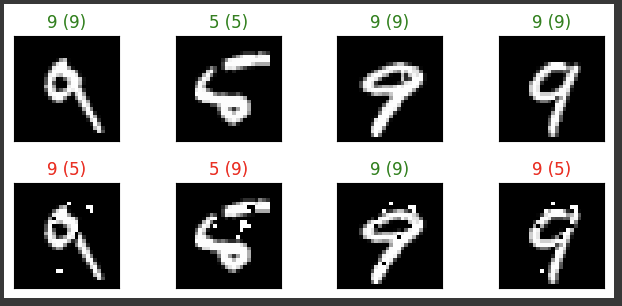As in flag 108.1, find all the correct predictions in the 25 images in the bottom row and concatenate their values to form the flag.

## Flag ML 108.4: Different Digits (10 pts)

Make these changes:
• Use 200 training samples
• Use 400 validation and test set samples
• Use the digits 1 and 2
• Use the perturbation type 12
• Use maximum perturbation 2.1
• Set the maximum iterations to 40
The first four modified images are shown below.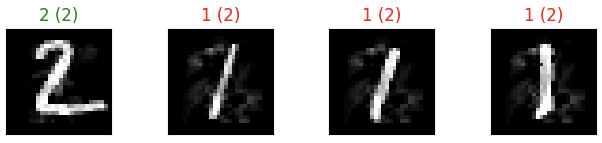As in flag 108.1, find all the correct predictions in the 25 images in the bottom row and concatenate their values to form the flag.

## Sources

https://github.com/pralab/secml/blob/master/tutorials/06-MNIST_dataset.ipynb